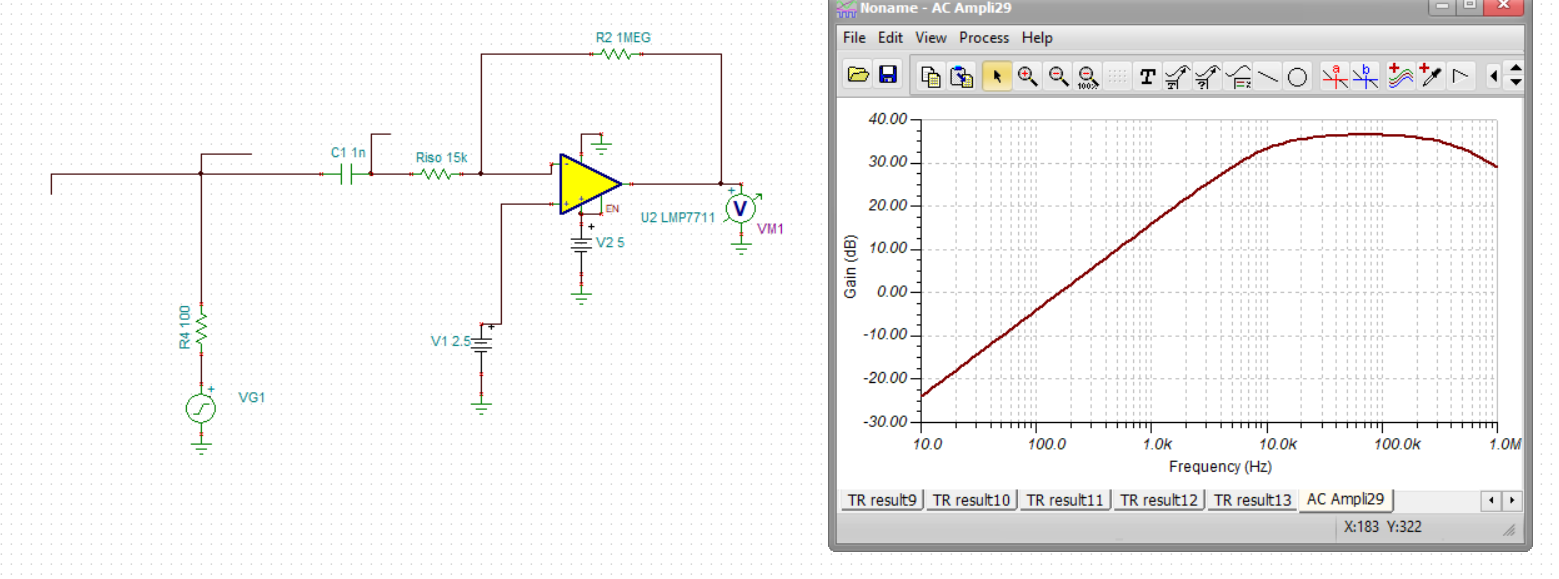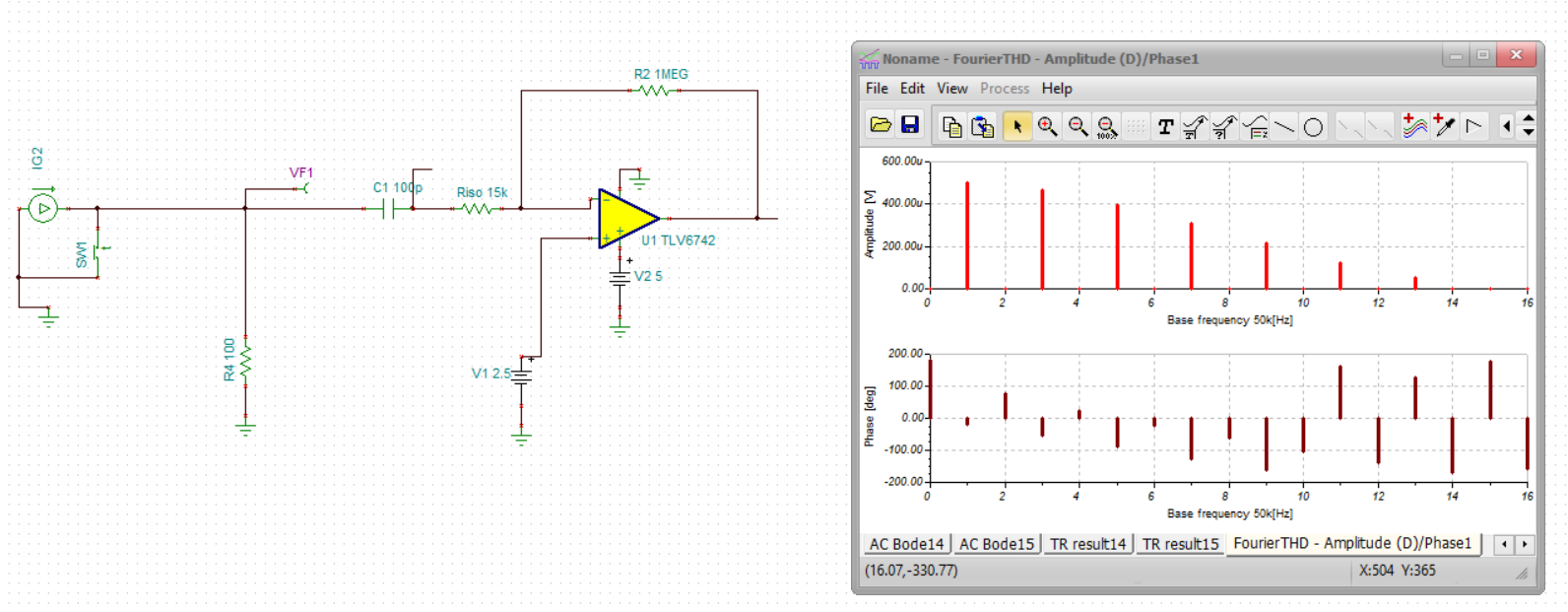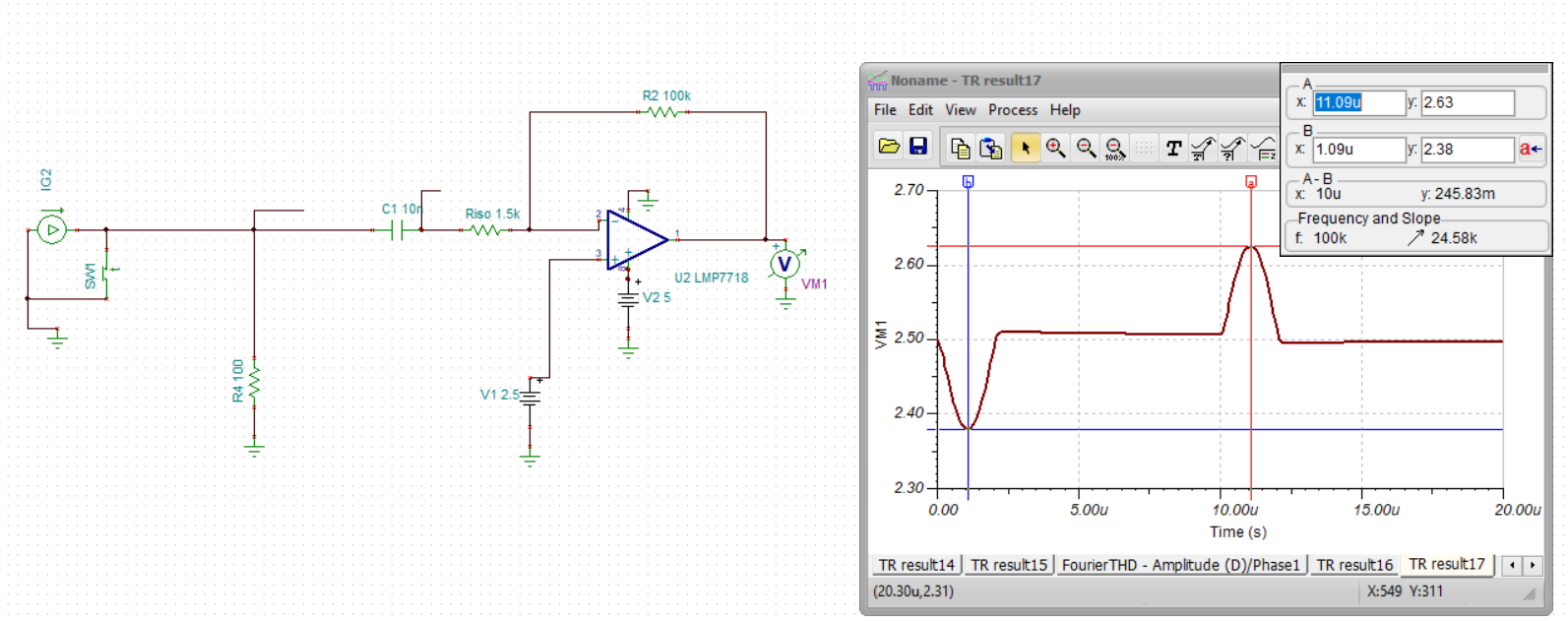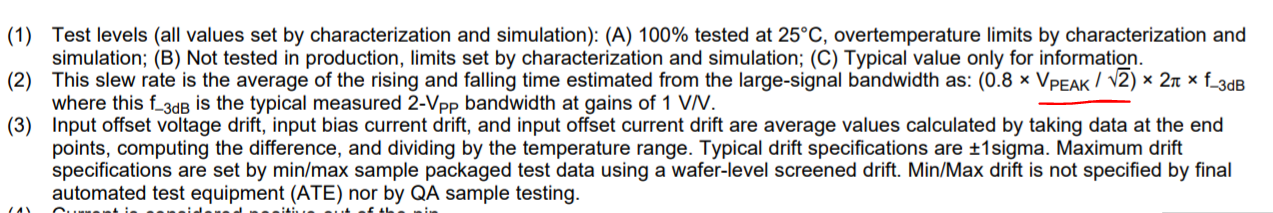• Resolved

# TLV6742: Slew rate and rise time of small signal

Part Number: TLV6742

Hi,

The slew rate of TLV6742 is lower than customer's requirements, however the input signal of customer's system is 2mV, which is small-signal. And from the precision lab training, the rise time of a small-signal step input is based on the op-amp’ s bandwidth and the rise time does not match up with the slew rate spec.

Questions:

1.  For customer's system:

f_c=GBW/G_cl =10MHz/48=208kHz

t_r=0.35/f_c =0.35/294kHz=1.68μs (Rise time)

Does it mean that no matter how much is the output voltage, the rise time is equal to 1.68μs?  But our customer requires the output to get to 3.3V within 1μs. Is the rise time in conflict with the requirement?

2. If the rise time does not match up with the slew rate spec, does it mean that i can choose an amp with arbitrary slew rate?

I'm confused by the rise time and the slew rate, and I really look forward to your reply.

Backup information:

Best regards,

Wendy

• Morning Wendy,

This is indeed a confusing area, where the precision labs repeats the simplified results in textbooks.

Your gain is high enough to ensure a single pole small signal response, What you care about on a step transition is not the average rise time but the peak dV/dT and compare that to the available slew rate. An ideal 1st order response has a peak dV/dT at time zero - of 1/tau. That never happens as all systems have higher poles that give you a dV/dt = 0 at time zero.

Up above your calculate a rise time of 1.68usec and then go off into a settling time discussion - those are different issues where you need many time constants (not just the 2.2X for rise time) to get to a final value.

So I think you need more GBP if 1usec settling (to what accuracy?) is needed. then, you can actually do a simulation of the intended device and circuit for the input time waveform, dump out the data into excel and run a point by point dV/dT to compare to the slew rate in the device - the models will often show slew limiting where the operation I just described will show a flat region in dV/dT if it hits the slew limit on transition.

I do have quite a bit of published material on this topic, but it is more 2nd order step response oriented and probably far beyond your immediate needs. Essentially, if the linear response produces a dV/dt profile on the output edges that never exceeds the available slew rate, then there response will stay linear.

Michael Steffes

• Hi Wendy,

Does it mean that no matter how much is the output voltage, the rise time is equal to 1.68μs?  If the rise time does not match up with the slew rate spec, does it mean that i can choose an amp with arbitrary slew rate?  I'm confused by the rise time and the slew rate, and I really look forward to your reply.

No.  Here is how I would separate the cases.  First, do the inputs separate significantly?  In other words, is the input step large?  If it is, then you will have the amplifier enter its slew rate region.  If not, then you are in the small signal region.

If you are in the small signal region, then your rise time is decided by the formula you have described.  The rise time will be the same regardless of the output, unless your output exceeds a certain voltage.  If the output amplitude goes too high, then the amplifier will be limited by the slew rate.  This is called the full-power bandwidth.  So, even if you stay in the small signal input region there is a limit to how much the output can change without distortion.  To avoid distortion, you need to operate within this curve.

This topic is explained in more detail in this section of TI Precision Labs.  Please let me know if you have any more questions.

Regards,

Daniel

• In reply to Michael Steffes:

Morning Michael and Daniel,

The input signal can be seen as half a sine wave of 2mVsin(500k*pi*t). However there is a bias, 2.5V. When i calculate the slew rate, shall i use the 2*pi*250kHz*(2mV*48), or shall I use the 2*pi*250kHz*(2mV*48+2.5)? And since there is a bias, 2.5V, can i regard the input signal to be small-signal?

And the circuit is attached here. How much is the slew rate enough for the circuit? Besides, the accuracy is within 1LSB=3.3V/2^12=0.8mV.slew rate.TSC

Thank you so much for your help.

Best regards,

Wendy

• In reply to Wenxiu Wang:

Thanks for the file Wendy, not really a slew limit issue, more of a BW issue on both the low and high side, attached - half wave amplifier tune up.docx

And here is the SSBW shape of that last ckt, much better flat region mid band,Michael Steffes

• In reply to Michael Steffes:

Hi Michael,

Thanks for the help here.  I walked right past the obvious answer and just gave a generic explanation instead.

Regards,

Daniel

• In reply to Wenxiu Wang:

This post was edited by the post author to reflect a correction to the final formula listed below.

Hi Wendy,

Michael is right.  The part does not have enough GBW.   Here's a simplified explanation. From simulation, your signal gain is about 67V/V.  So, the noise gain is 67V/V + 1V/V = 68V/V.  Divide the GBW of the amplifier (10Mhz) by the noise gain (68V/V) and you get a circuit bandwidth of 147kHz.  This matches the simulation value, by the way.

Your signal must be less than 147kHz and in real life you want to leave some significant margin.  If we look at your input waveform, we can approximate it as a half sine-wave lasting 2us or a sine wave with a period of 4us.  This corresponds to a signal frequency of 250kHz which is faster than our amplifier can provide at this gain.  So, you will need a faster part (more GBW).  Again, the signal may not be a pure sine wave.  This is just an approximation.

I would also like to point out a couple other things.  If you are not bandwidth or slew rate limited, then the rise time is the same for any small signal.  But, you need to take into account that this is not the settling time.  The rise time is how long it takes to go from 10% to 90% of the final output value.  Settling time will be longer and can depend on the stability of the amplifier.

Lastly, when calculating the necessary slew rate I would use this formula: SR > 2 * pi * (250kHz) * (output signal magnitude).  I would not worry about any offset voltage, but just the magnitude of the output wave.  We are trying to measure how fast the amplifier can respond.  Note that the formula is valid for sinusoidal signals.
Regards,
Daniel
• In reply to Daniel Miller56:

Thanks, once there is a starting TINA file, I get a more interested.

Michael Steffes

• In reply to Michael Steffes:

Another way to think about your required BW is how many spectral components of the Input fourier series do you want to pass. The input here is 50kHz, it mainly has odd harmonics, so if you want to pass the first 4 odd harmonics you need have a flat response through the 9th*50kHz or 450kHz. At a gain of 66, that is a 30MHz GBP.The LMP7718 is a decomp 88MHz GBP, I had to reduce the R's (increasing the input C to 10nF) to get rid of some ringing, but now the output Vpp is exactly as expected for this 1.3MHz BW solution,Michael Steffes

• In reply to Daniel Miller56:

Also Daniel, in reading this reply way at the end you calculate a required SR by the 2piFmaxVpp. Actually  -

1. This classic equation taking the derivative at zero crossing uses the Vpeak not Vpp. And

2. This classic equation has always been wrong, when you measure the F-3dB in a LSBW test, are you not -3dB on the measurement (again, 3dB down on the fundamental in a Fourier series). So there should also be a 0.707 factor time Vpeak.

I had started changing the footnote relating SR to FPBW to include that factor way back in the late 80's. But apparently, I am the only one - for instance, from the OPA837 spec tables - This one included a 0.8X factor from an app note Xavier Ramus did - kind of an empirical adjustment - for modern devices, this mapping is only approximate.That app note is one of the references in this pair of more recent articles to map SSBW shapes to peak dV/dT and how to extract slew rate from LSBW plots (in part 2).www.edn.com/.../

Michael Steffes

• In reply to Michael Steffes:

Hi Michael,

1.  Thanks for catching that.  I've edited my response to show the correction.  Funnily enough, I referenced the "magnitude of the output wave" in the very next sentence.

2.  What does the "W" in "LSBW" stand for?  I've yet to hear this theory.  I suppose that if you have to multiply Vpeak by a factor of 2^(1/2), this would just give you a more optimistic slew rate requirement or peak voltage capability.

Regards,
Daniel